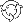The Theory \ Details \ general information \ prices correction

# General Information

## Correction of historical prices

There are several cases when the price of an asset changes while its value for an investor stays the same. In such situations it is necessary to impose corrections to the corresponding time series. Correction is necessary in the case of the following events:
• Dividend payments in Stocks and Mutual Funds
• Splits and Reversed Splits in Stocks and Mutual Funds
• Rollovers in Futures

The general correction algorithm is described below.
Imagine that there is a gap on the price chart, which is induced by one of the above-listed events. Let old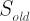and new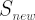denote the prices just before and after the break point respectively.Then the correction coefficientis calculated by the following formula:. All the prices before the break point are then multiplied by.

In case of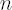gaps let’s start by sorting them from the latest to the earliest. The corresponding correction coefficients denote by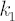,...,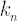.

Let’s multiply byall the prices that lie between the second and the first break points. Then multiply the prices between the third and the second break points by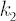. Subsequently performing the analogous
operation over all other time intervals bounded by adjacent break points, we will finish at the section that lies to the left of the earliest break point. It is easy to see that its prices must be multiplied by.